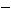Subtract Fractions and Mixed Numbers

Subtract Fractions and Mixed Numbers
Fractions with Common Denominators

Fractions with the same denominator are subtracted by placing the difference of the numerators over the common denominator.  After subtracting, write the fraction in simplest form (if necessary).

Fractions with Different Denominators

Find the least common denominator (LCD) of the fractions.

• The common denominator is the least common multiple (LCM) of the original denominators.
• Rewrite the fractions as equivalent fractions with that LCM as their denominators.
• Subtract.

Example

The least common multiple of 10 and 20 is 20, then

The lowest common denominator (LCD) of the fractions is 20, then

Simplify the difference (not needed in this example)

Mixed Numbers

To subtract mixed numbers without borrowing, subtract the fractional parts and then subtract the whole numbers.

Subtraction of mixed numbers may involve borrowing

• To borrow "1" from a whole number, reduce the whole number by 1 and add 1 to the fractional part.
• Rewrite the borrowed "1" as a fraction with the same common denominator as the fractional part (to have a value of "1," that fraction needs the same numerator and denominator)

Example

Find 51 2/3.

Rename 5 as 4 + 1, and then rename 1 using 3 as a denominator (Any fraction with the same numerator and denominator is equivalent to 1)

Subtract the fractions

Subtract the whole numbers

41 = 3

The result is

Example

First, rename the fractions so they have common denominators:

Since we can't take 12 from 5, we clearly need to borrow.  Rename 6 as 5 + 1, then rename 1 as 15/15.  This gives us